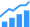# Making Accurate Power Estimates At RTL

The key is understanding where power is used and combining all the ingredients. Here’s the list.It may seem counterintuitive, but an accurate estimation of power at Register Transfer Level can be made. In this blog, we will learn how it can be done.

The main ingredient
In order to understand RTL power estimation, let us first consider making the power estimation at gate level. At gate level we have a netlist that contains standard cell instances. These standard cells have been characterized for (among other things) their power dissipation. The Liberty file for the relevant library contains power numbers for every cell in the netlist and a wire load model.

The power values in the Liberty file are for static and dynamic power. The static power numbers are the leakage for each cell. The dynamic power consists of short-circuit power and switching power.

Short circuit (aka crow bar power) is the power that is dissipated during switching when both the N and P channel transistors are on. This causes a (hopefully) brief current flow path from rail to rail, which is why it is called short circuit current. Short circuit power can vary based on the input slew rate.

Switching power (aka capacitive power) is the power used to change the logic state. It involves charging or discharging the internal capacitance of the driving cell, the input capacitance of the driven cells, and the interconnect wire capacitance. To calculate this type of power, we need these various capacitance values. The liberty file contains the cell capacitances, but not the interconnect capacitances.

Interconnect capacitance can be measured from a layout using a layout extraction tool. That is the most accurate method. Interconnect capacitances can also be estimated from a wire load model if the layout is not yet available. The wire load models are often included in the Liberty files.

Switching activity
Switching a logic state requires a certain amount of energy. This is the energy associated with charging (or discharging) a capacitor. To calculate the switching power, we need the energy for a single switching event and the rate of the switching. The switching rate is expressed as the product of the clock frequency times the toggle density. Toggle density is the number of switching events per clock cycle.

Toggle density is generally obtained through simulation. The power estimate will be for the scenario that was simulated. The selected scenario(s) could represent a typical use case, or the scenario might be a corner case for maximum power.

Clock tree
In most designs, the clock tree is a significant contributor to the overall power. It often accounts for more than half of the total power. If the layout is complete, we can get the clock tree information from the netlist written by the layout tool and the capacitances can be extracted from the layout itself.

Operating condition
The final ingredient is the operating condition. The power will vary based on the supply voltage, temperature, and process variation. These values are used to select the correct values from the Liberty file.

Putting it all together
Getting the power estimate is straightforward when all of the ingredients are available. The power will be the sum of the powers for each of the cells instantiated in the netlist.

Power estimation at RTL
At the Register Transfer Level, some ingredients are missing, particularly the gate level netlist and the layout. Also, there is no clock tree information.

For an RTL power estimation tool, we would need to use a logic synthesis engine to create a gate level model of the design. From this we can apply the same techniques as for the gate level flow.

That leaves the clock tree. In order to estimate the clock tree power, we need some information from the layout engineer. We need to know which buffers will be used and what their fan-outs will be. One method of estimating the clock tree is this. From the netlist, we can get the number of flip flops per clock domain. We can now calculate the number of buffers driving the flip flops, i.e. the number of flip flops divided by the fanout is the number of final clock buffers. We can repeat the process to get the rest of the clock tree. But this is not the most accurate method.

Improving power estimation accuracy at RTL
Power estimation accuracy can be improved by several methods. One method is to create a more accurate wire load model. If the design in question is not the first design in this technology, a lot can be learned from an existing layout. It is straightforward, for example, to extract a wire load model from a layout. A SPEF file contains the necessary information.

Another way to improve accuracy, particularly at newer process nodes, is to handle the use of multiple libraries. It is common practice to use several libraries with different speed-power tradeoffs. This is done by changing the implants to adjust the threshold voltages of the transistors (Vth). A lower Vth device conducts more current both in terms of leakage and dynamic power. The key is to know how often the low Vth cells are used and factor that into the power calculations.

A more accurate method for getting the clock tree power is to perform clock tree synthesis, just as the layout tool creates the clock tree. After the clock tree is created, power can be estimated in the usual way.

Current process nodes have very resistive wires, which require buffering when they are long. In practice, these added buffers increase overall power slightly. Modeling this can provide additional accuracy.

Conclusion
Power can be estimated with surprising accuracy at RTL. A number of techniques were described which are used to accomplish this.